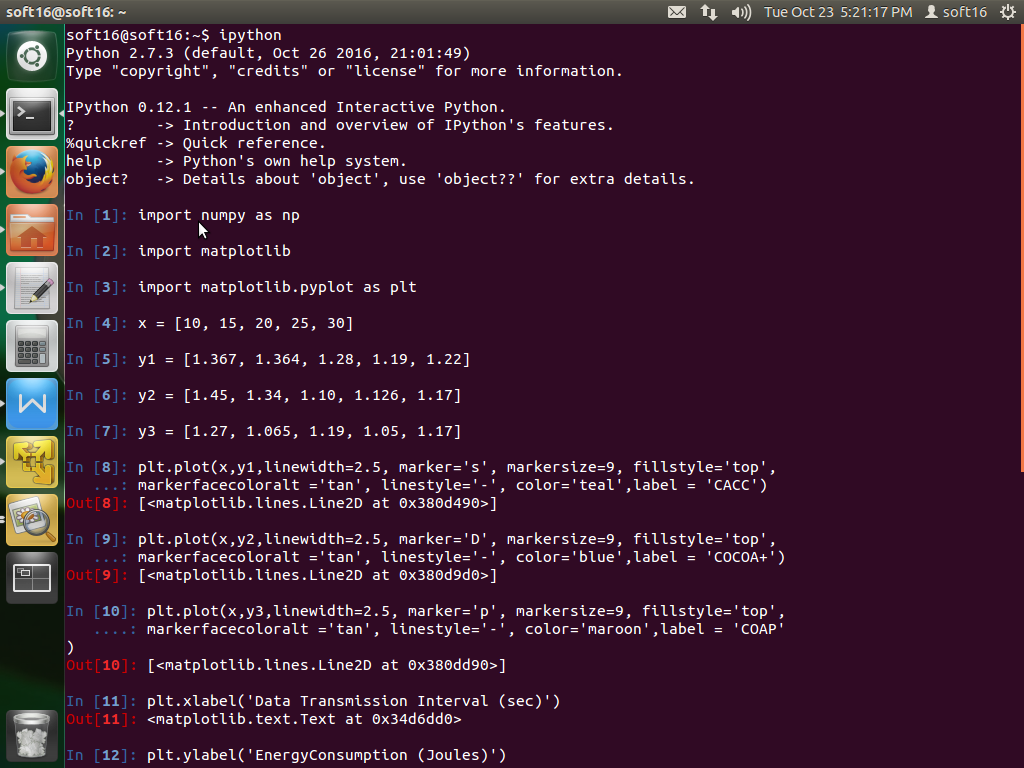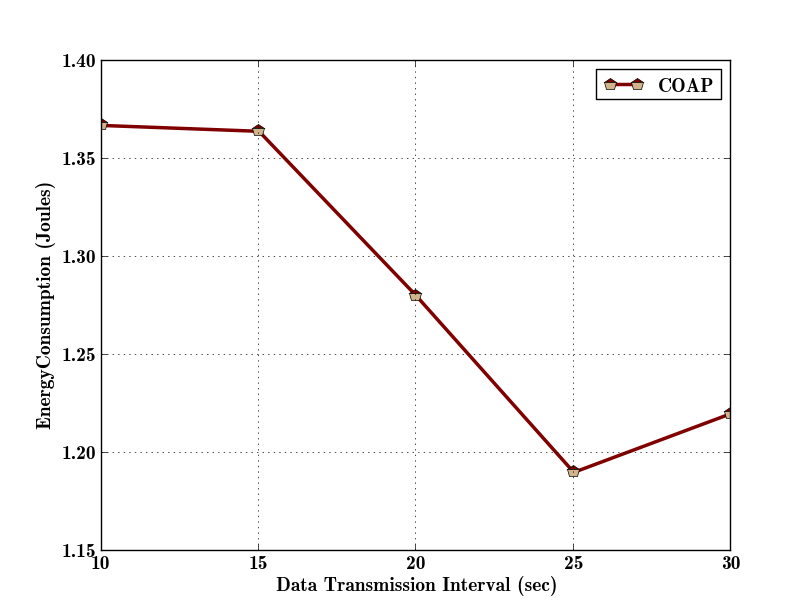• #5, First Floor, 4th Street Dr. Subbarayan Nagar Kodambakkam, Chennai-600 024 Landmark : Samiyar Madam
• pro@slogix.in
• +91- 81240 01111

#### Social List

##### How to calculate the Energy consumption of CoAP message during transmission using cooja simulator?
###### Description

Energy consumption is the amount of energyor powerused to transmit or receive data packets.

###### Step-1

Open file to modify to calculate

energy consumption at following location:

home/ contiki/ examples/er-rest-

examples/er-example-server.c

Code:

Edit and use following code inside a Response handler function

unsigned long cpu_time, lpm_time, rx_time, tx_time;

lpm_time = energest_type_time

(ENERGEST_TYPE_LPM);

cpu_time = energest_type_time

(ENERGEST_TYPE_CPU);

rx_time = energest_type_time

(ENERGEST_TYPE_LISTEN);

tx_time = energest_type_time

(ENERGEST_TYPE_TRANSMIT);

unsigned long energy_consumed;

unsigned long t1;

t1 = 1.8 * cpu_time ;

unsigned long t2;

t2 = 0.0545 * lpm_time;

unsigned long t3;

t3 = 20.0 * rx_time;

unsigned long t4;

t4=17.7*tx_time;

unsigned long t11;

unsigned long t22;

unsigned long t33;

t11 = (20.0 * rx_time + 17.7 * tx_time ) * 3 ;

t22 = (unsigned long)

(((1.8 * cpu_time + 0.0545 * lpm_time + 20.0

* rx_time + 17.7 Code: * tx_time ) * 3) /

RTIMER_SECOND);

t33 = (unsigned long) (((t22 *

(1.8 * cpu_time + 0.0545 * lpm_time)) * 3)

/ RTIMER_SECOND);

power_consumed = (unsigned long)

(t11 / RTIMER_SECOND);

printf(“Energy CONSUMPTION IS %lu ID

%d\n”,t33, node_id); // take performance

}

###### Open Cooja:

Open contiki cooja network
simulator and create new
simulation(ctrl+n).

rpl-border-router.c, er-
example-server.c, er-example-client.c
create motes for

Server ,client location:
home/contiki/examples/
er-rest-example.

Border router location:
home/contiki/examples/ipv6/
rpl-border-router

create new motes type

Connect the router to server
.In terminal at above location.

make-connect-router-cooja

Code for to calculate energy
consumption modify in existing (.csc)
script file before re-simulation.

if(msgArray.equals(“Energy”))
{
if(msgArray.length == 6)
{
e_count++;
energy_t = parseInt(msgArray);
totalenergy = energy_t + totalenergy;
energyconsumeinticks =
totalenergy / e_count;
//now unit in milliwatts/ticks=
1milliwats=0.001 joules
energyconsumption=
energyconsumeinticks / 1000;
energyconsumption =
energyconsumption / 128;
log.log(” Energy_consumption ”
+ energyconsumeinticks +
” energyconsumption ” + energyconsumption
+ ” Joules” + “\n”);
}
}We can see energy consumption calculating in script editor output.

###### Step-3

Performance evaluation

Code:

import numpy as np

import matplotlib

import matplotlib.pyplot as plt

x = [10, 15, 20, 25, 30]

y1 = [1.367, 1.364, 1.28, 1.19, 1.22]

plt.plot(x,y1,linewidth=2.5,

marker=’p’, markersize=9,

fillstyle=’top’,

markerfacecoloralt =’tan’,

linestyle=’-‘, color=’maroon’,label = ‘COAP’)

plt.xlabel

(‘Data Transmission Interval (sec)’)

plt.ylabel

(‘EnergyConsumption (Joules)’)

plt.rc

(‘font’,family=’Times New Roman’)

plt.rcParams.update

({‘font.size’:14})

plt.legend(loc=’center ‘,

prop={‘size’:14})

plt.grid()

plt.show()

Open terminal >>ipython >>

enter codes >> enter.###### Step-4

After compilation enter to plot the graph and save it.